# Physics - Classical Mechanics - Simple Pendulum

in STEMGeeks5 months ago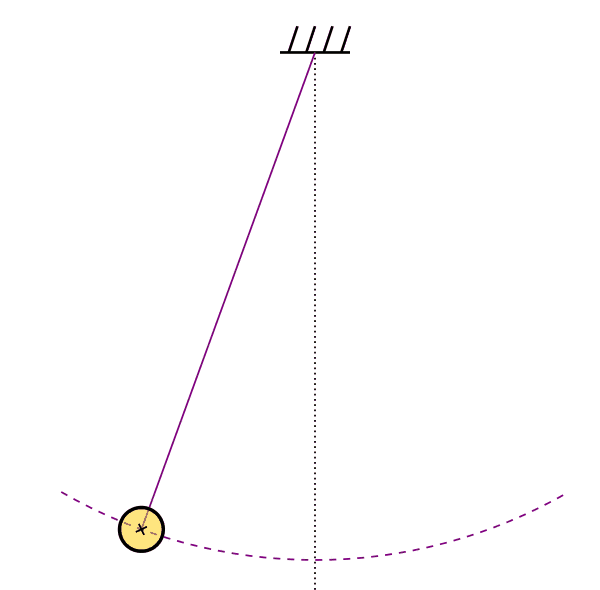[Image1]

## Introduction

Hey it's a me again @drifter1 with the first post of the year 2022! I hope it started great for all of you, and best wishes for the new year!

Today we continue with Physics, and more specifically the branch of "Classical Mechanics" in order to get into Simple Pendulums.

So, without further ado, let's get straight into it!

## Simple Pendulum

Periodic motion is not limited to simple harmonic motion (SHM) that we covered up to this point! Of course, not all periodic motion systems have to contain springs.

The simple pendulum is yet another idealized model for periodic motion. In such a system, a point mass m is hanging from a weight-less and non-extensible string within a homogeneous gravity field. Moving the mass from the equilibrium position to one side causes an oscillation (or periodic motion) around the equilibrium position.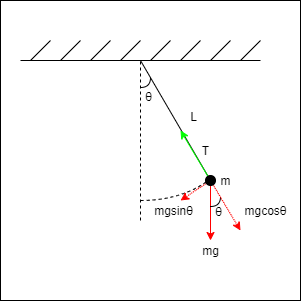### Return Force

The oscillation "route" in the case of a pendulum is not linear, but a circle arc. An arc from a circle with a radius equal to the length of the string, commonly denoted L.

If θ is the angular displacement from the vertical equilibrium position, then the component of the gravitational force that acts upon that route, and so the return force, is given by: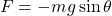This also proves that such a periodic motion is not simple harmonic motion, as the return force is not proportional to x or at least θ, but instead sinθ.

### Small Angle Approximations

When θ is very small, we can approximate sinθ with θ, which gives us: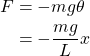and so an equation of the form F = -kx, where mg/L plays the role of the k spring constant.

This allows us to approximate pendulum oscillation as if it was simple harmonic motion (!) and gives us equations such as: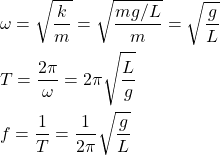These equations don't dependent on the mass of the object, but only of the length of the string, L.

Using these, we could also approximate the angular displacement as: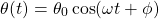### More Accurate Period

Let's also mention how one would approach getting more accurate results! The period can be expressed as an infinite series of the form: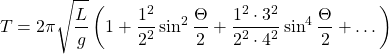where Θ is the maximum angular displacement (basically the amplitude).

For example, for Θ = 15° the difference between the approximation and this series is only about 0.5%, so the approximation can be considered quite sufficient!

### Gravity Approximation

It makes sense to use a pendulum in order to approximate the acceleration of gravity, as the period and length can be calculated easily and with great accuracy!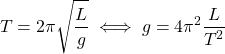## Final words | Next up

And this is actually it for today's post!

Next time we will cover Physical Pendulums...

See ya!Keep on drifting!

Posted with STEMGeeks

Sort:Thanks for your contribution to the STEMsocial community. Feel free to join us on discord to get to know the rest of us!

Please consider delegating to the @stemsocial account (85% of the curation rewards are returned).

You may also include @stemsocial as a beneficiary of the rewards of this post to get a stronger support.

Wow!... brings back memories.
Thanks for this.